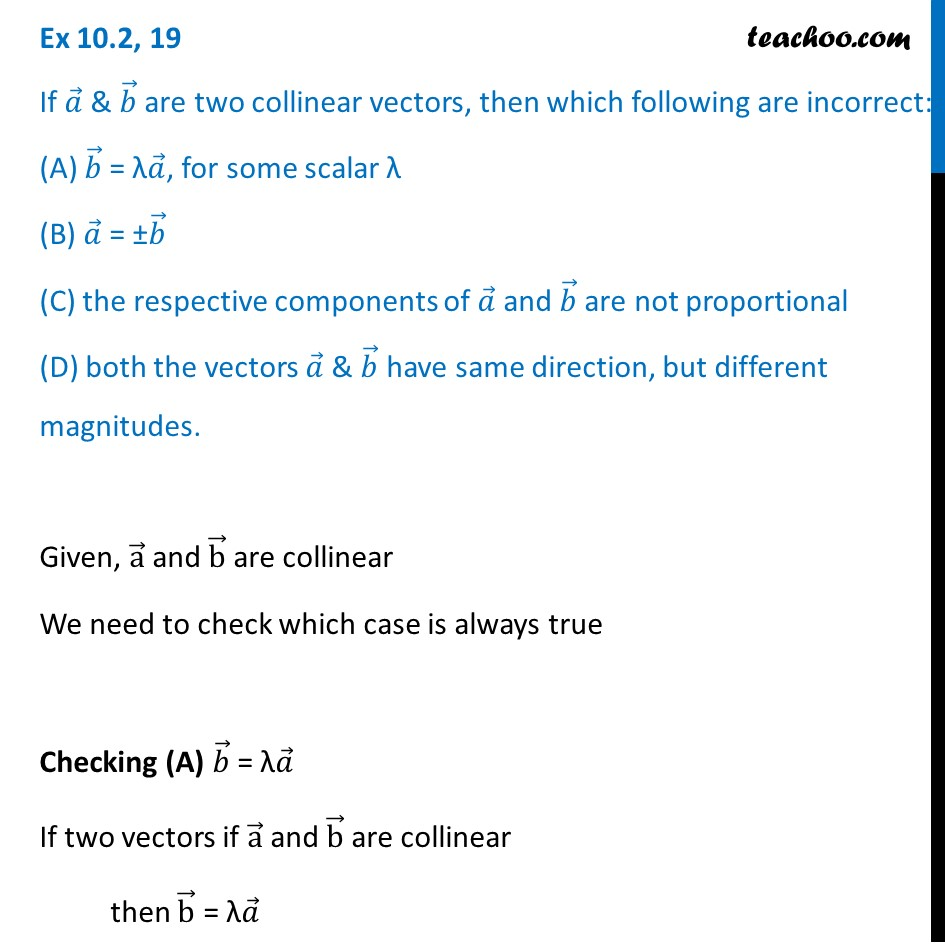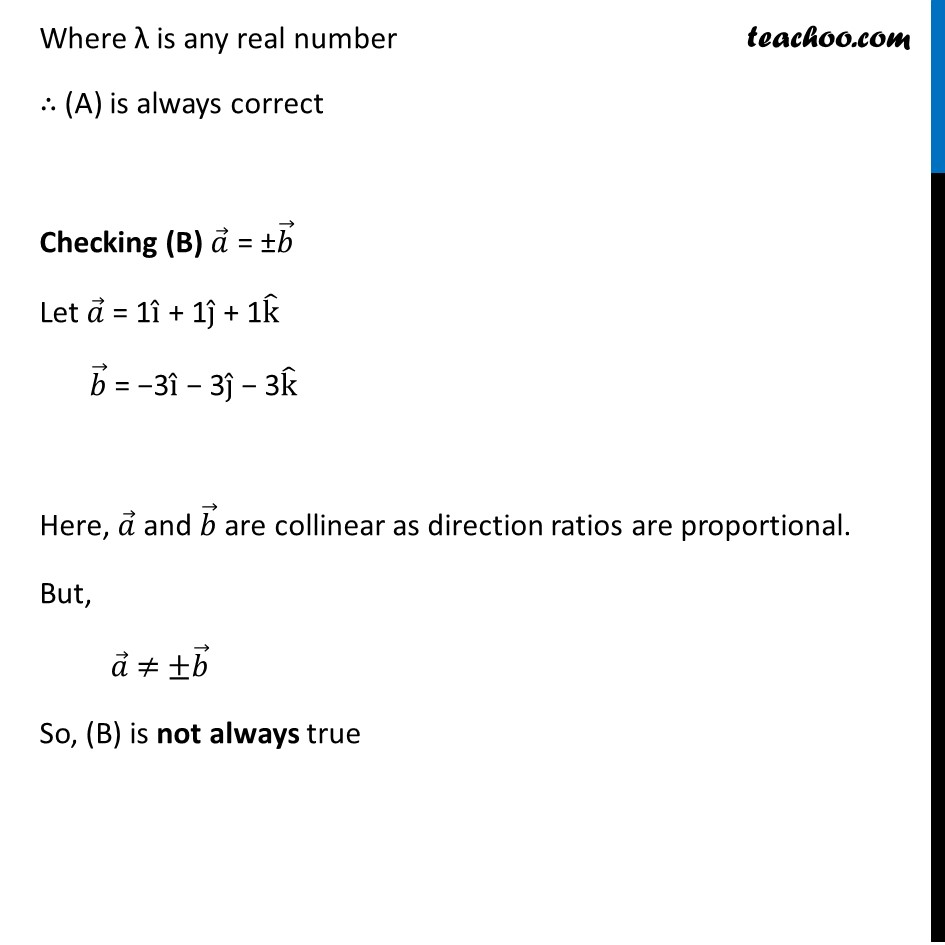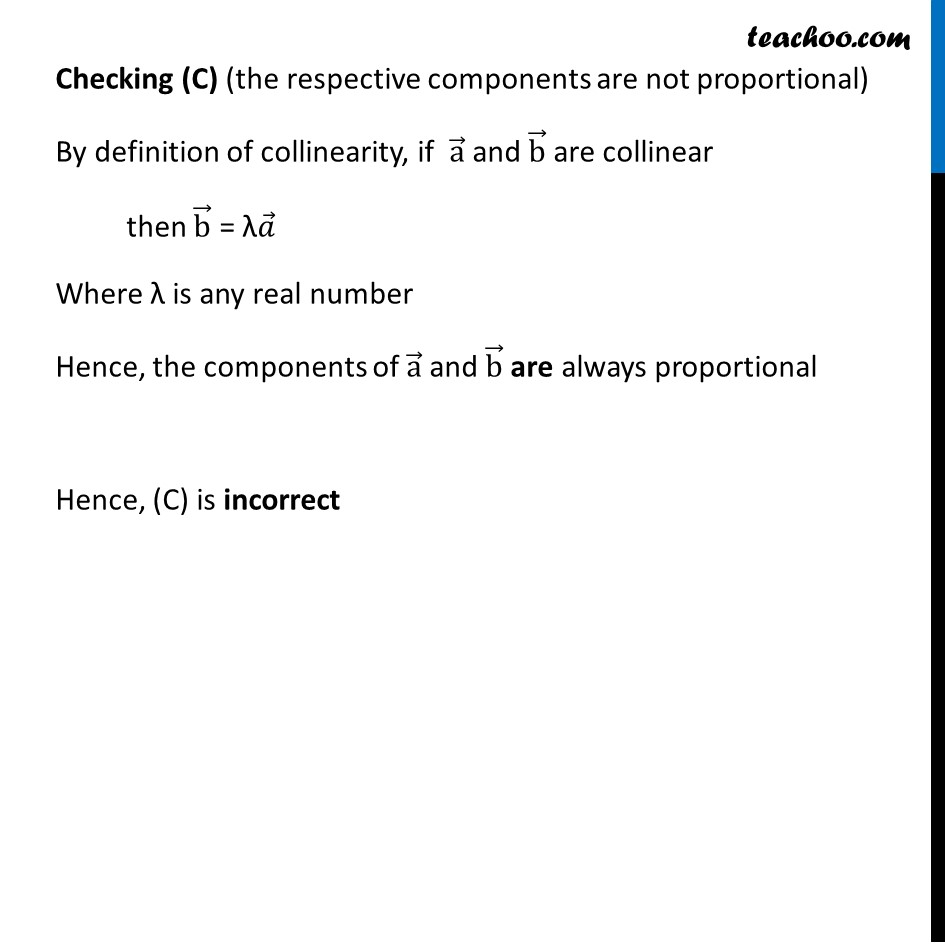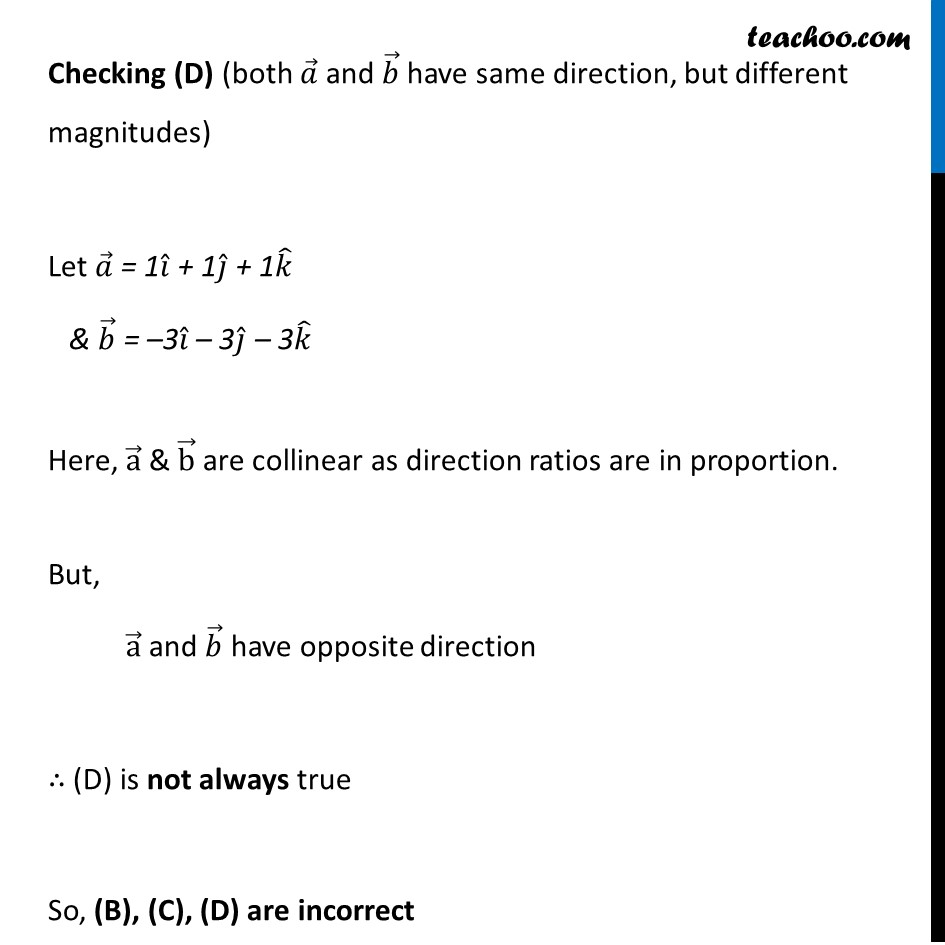Ex 10.2

Chapter 10 Class 12 Vector Algebra
Serial order wiseLearn in your speed, with individual attention - Teachoo Maths 1-on-1 Class

### Transcript

Ex 10.2, 19 If 𝑎 ⃗ & 𝑏 ⃗ are two collinear vectors, then which following are incorrect: (A) 𝑏 ⃗ = λ𝑎 ⃗, for some scalar λ (B) 𝑎 ⃗ = ±𝑏 ⃗ (C) the respective components of 𝑎 ⃗ and 𝑏 ⃗ are not proportional (D) both the vectors 𝑎 ⃗ & 𝑏 ⃗ have same direction, but different magnitudes. Given, a ⃗ and b ⃗ are collinear We need to check which case is always true Checking (A) 𝑏 ⃗ = λ𝑎 ⃗ If two vectors if a ⃗ and b ⃗ are collinear then b ⃗ = λ𝑎 ⃗ Where λ is any real number ∴ (A) is always correct Checking (B) 𝑎 ⃗ = ±𝑏 ⃗ Let 𝑎 ⃗ = 1i ̂ + 1j ̂ + 1k ̂ 𝑏 ⃗ = −3i ̂ − 3j ̂ − 3k ̂ Here, 𝑎 ⃗ and 𝑏 ⃗ are collinear as direction ratios are proportional. But, 𝑎 ⃗ ≠ ±𝑏 ⃗ So, (B) is not always true Checking (C) (the respective components are not proportional) By definition of collinearity, if a ⃗ and b ⃗ are collinear then b ⃗ = λ𝑎 ⃗ Where λ is any real number Hence, the components of a ⃗ and b ⃗ are always proportional Hence, (C) is incorrect Checking (D) (both 𝑎 ⃗ and 𝑏 ⃗ have same direction, but different magnitudes) Let 𝑎 ⃗ = 1𝑖 ̂ + 1𝑗 ̂ + 1𝑘 ̂ & 𝑏 ⃗ = –3𝑖 ̂ – 3𝑗 ̂ – 3𝑘 ̂ Here, a ⃗ & b ⃗ are collinear as direction ratios are in proportion. But, a ⃗ and 𝑏 ⃗ have opposite direction ∴ (D) is not always true So, (B), (C), (D) are incorrect